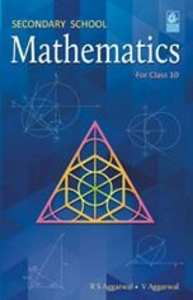Share

# R.S. Aggarwal solutions for Class 10 Mathematics chapter 6 - T-Ratios of some particular angles

## Secondary School Mathematics for Class 10 (for 2019 Examination)

#### R.S. Aggarwal Secondary School Mathematics Class 10 (for 2019 Examination)## Chapter 6: T-Ratios of some particular angles

#### Chapter 6: T-Ratios of some particular angles solutions [Page 0]

Evaluate:
sin600 cos300 + cos600 sin300

Evaluate:
cos600 cos300− sin600 sin300

Evaluate:
cos450 cos300 + sin450 sin300

Evaluate:
(sin30°)/(cos 45°)+(cot45°)/(sec60° )- (sin60°)/(tan45°)+(cos30°)/(sin90°)

Evaluate:
(5 cos^2 60^0 + 4 sec^2 30^0 -tan^2 45^0)/(sin^2 30^0+cos^2 30^0)

Evaluate:

2cos^2 60^0+3 sin^2 45^0 - 3 sin^2 30^0 + 2 cos^2 90 ^0

Evaluate:

cot^2 30^0-2cos^2 30^0-3/4 sec^2 45^0 +1/4 cosec^2 30^0

Evaluate:

(sin^2 30^0 + 4 cot^2 45^0-sec^2 60^0)(cosec^2  45^0 sec^2 30^0)

Evaluate:

4/(cot^2 30^0) +1/(sin^2 30^0) -2 cos^2 45^0 - sin^2 0^0

Show that:

(i) (1-sin 60^0)/(cos 60^0)=(tan60^0-1)/(tan60^0+1)

Show that:
(ii) (cos30^0+sin 60^0)/(1+sin30^0+cos60^0)=cos 30^0

Verify each of the following:

(i)sin 60^0 cos 30^0-cos 60^0 sin 30^0

Verify each of the following:

(ii)cos 60^0 cos 30^0+ sin 60^0 sin30^0

Verify each of the following:

(iii) 2 sin 30^0 cos 30^0

Verify each of the following:

(iv) 2 sin 45^0 cos 45^0

If A = 450, verify that :
(i) sin 2A = 2 sin A cos A

If A = 45, verify that:

(ii) cos 2A = 2 cos2 A – 1 = 1 – 2 sin2

If A = 30, verify that:

(i) sin 2A = (2 tan A)/(1+tan^2A)

If A = 30, verify that:

(ii) cos 2A = (1- tan^2A)/(1+tan^2A)

If A = 30, verify that:

(iii) tan 2A = (2tanA)/(1-tan^2A)

If A = 600 and B = 300, verify that:
(i) sin (A + B) = sin A cos B + cos A sin B

If A = 600 and B = 300, verify that:

cos (A + B) = cos A cos B - sin A sin B

If A = 600 and B = 300, verify that:
(i) sin (A – B) = sin A cos B – cos A sin B

If A = 600 and B = 300, verify that:

(ii) cos (A – B) = cos A cos B + sin A sin B

If A = 600 and B = 300, verify that:

(iii) tan (A-B) = (tan A-tanB)/(1+tan A tan B)

If A and B are acute angles such that tan A =1/3, tan B = 1/2  and tan (A + B) = show that  A+B = 45^0

Using the formula, tan 2A =(2 tan A )/(1- tan^2 A) find the value of tan 600, it being given that tan 300 = 1/sqrt(3).

Using the formula, cos A = sqrt((1+cos2A)/2) ,find the value of cos 300, it being given that cos 600  = 1/2.

Using the formula, sin A = sqrt((1-cos 2A)/2)  find the value of sin 300, it being given that cos 600 = 1/2

In the adjoining figure, ΔABC is a right-angled triangle in which ∠B = 900, ∠300 and AC = 20cm. Find (i) BC, (ii) AB.

In the adjoining figure, ΔABC is right-angled at B and ∠A = 300. If  BC = 6cm, find (i) AB, (ii) AC.

In the adjoining figure, ΔABC is right-angled at B and ∠A = 450. If AC = 3sqrt(2)cm, find (i) BC, (ii) AB.

If sin (A + B) = 1 and cos (A – B) = 1, 0 ≤ (A + B) ≤ 900  and A > B, then find A and B.

If sin (A – B) = 1/2 and cos (A + B) = 1/2, 0^0 ≤ (A + B) ≤ 90^0 and A > B, then find A and B.

If tan (A – B) = 1/sqrt(3) and tan (A + B) = sqrt(3), 0^0 ≤ (A + B) ≤ 90^0 and A > B, then find A and B.

If 3x = cosecθ = and 3/x= cottheta find the value of 3(x^2-1/x^2).

If sin (A+B) = sin A cos B + cos A sin B and cos (A-B) = cos A cos B + sin A sin B
(i) sin (750)
(ii) cos (150

## Chapter 6: T-Ratios of some particular angles

#### R.S. Aggarwal Secondary School Mathematics Class 10 (for 2019 Examination)## R.S. Aggarwal solutions for Class 10 Mathematics chapter 6 - T-Ratios of some particular angles

R.S. Aggarwal solutions for Class 10 Maths chapter 6 (T-Ratios of some particular angles) include all questions with solution and detail explanation. This will clear students doubts about any question and improve application skills while preparing for board exams. The detailed, step-by-step solutions will help you understand the concepts better and clear your confusions, if any. Shaalaa.com has the CBSE Secondary School Mathematics for Class 10 (for 2019 Examination) solutions in a manner that help students grasp basic concepts better and faster.

Further, we at Shaalaa.com provide such solutions so that students can prepare for written exams. R.S. Aggarwal textbook solutions can be a core help for self-study and acts as a perfect self-help guidance for students.

Concepts covered in Class 10 Mathematics chapter 6 T-Ratios of some particular angles are Introduction to Trigonometry, Introduction to Trigonometry Examples and Solutions, Trigonometric Ratios, Trigonometric Ratios of an Acute Angle of a Right-angled Triangle, Trigonometric Ratios of Some Specific Angles, Trigonometric Ratios of Complementary Angles, Trigonometric Identities, Proof of Existence, Relationships Between the Ratios.

Using R.S. Aggarwal Class 10 solutions T-Ratios of some particular angles exercise by students are an easy way to prepare for the exams, as they involve solutions arranged chapter-wise also page wise. The questions involved in R.S. Aggarwal Solutions are important questions that can be asked in the final exam. Maximum students of CBSE Class 10 prefer R.S. Aggarwal Textbook Solutions to score more in exam.

Get the free view of chapter 6 T-Ratios of some particular angles Class 10 extra questions for Maths and can use Shaalaa.com to keep it handy for your exam preparation

S